# WHAT ARE THE PERIODIC TRENDS Periodic Trends All

• Slides: 13WHAT ARE THE PERIODIC TRENDS?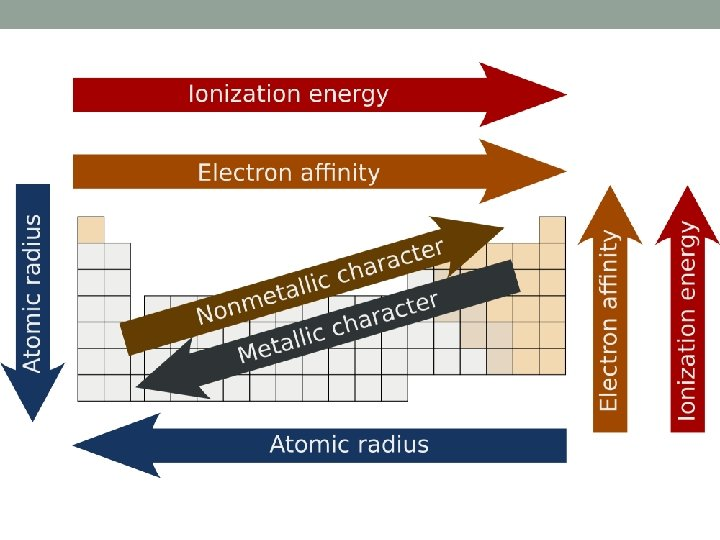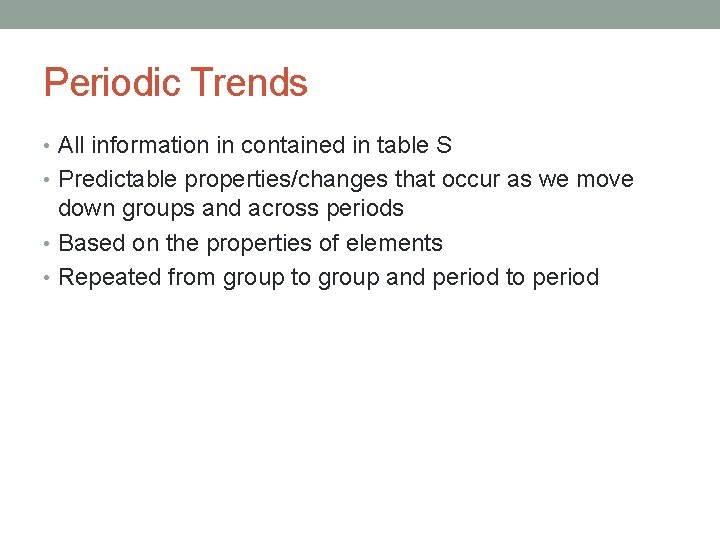Periodic Trends • All information in contained in table S • Predictable properties/changes that occur as we move down groups and across periods • Based on the properties of elements • Repeated from group to group and period to period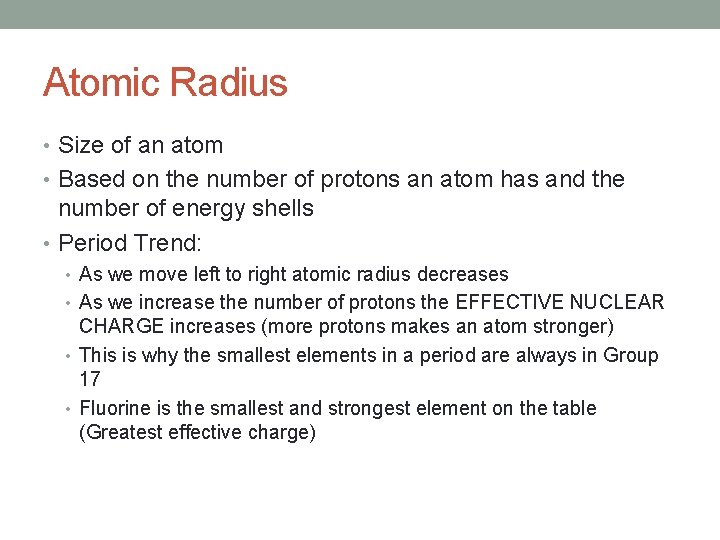Atomic Radius • Size of an atom • Based on the number of protons an atom has and the number of energy shells • Period Trend: • As we move left to right atomic radius decreases • As we increase the number of protons the EFFECTIVE NUCLEAR CHARGE increases (more protons makes an atom stronger) • This is why the smallest elements in a period are always in Group 17 • Fluorine is the smallest and strongest element on the table (Greatest effective charge)Atomic Radius Cont: • Group Trend: • As we move down a group atomic radius INCREASES • This is because the number of energy levels increases • Each level adds a shell and so the atom occupies more volume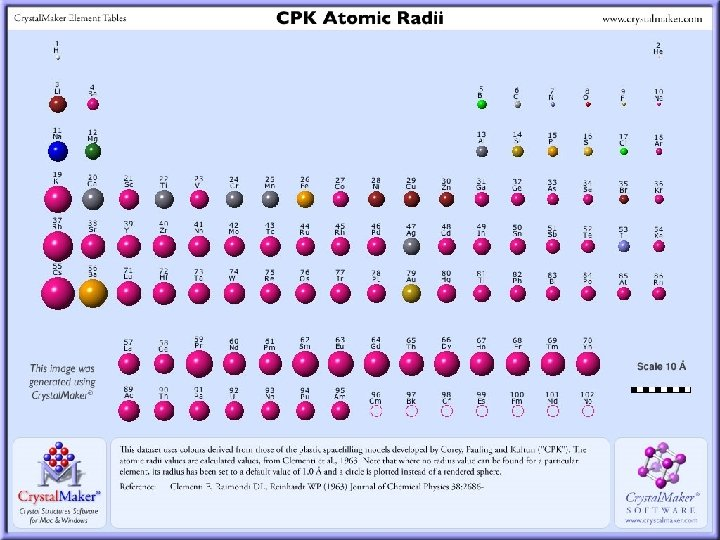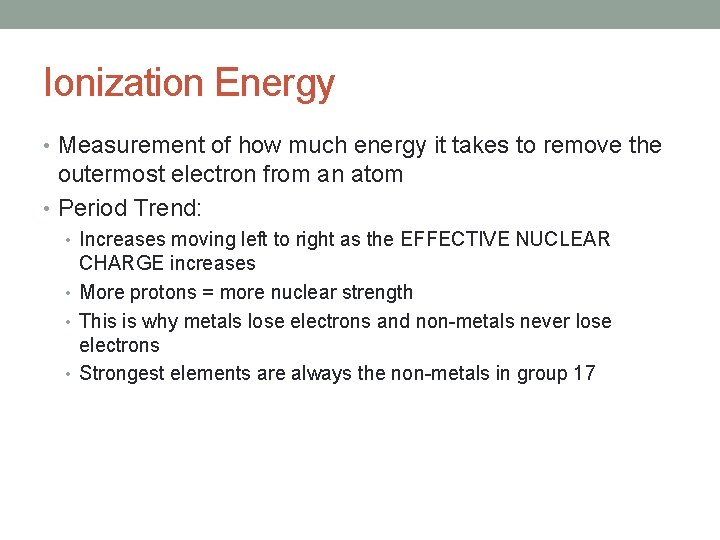Ionization Energy • Measurement of how much energy it takes to remove the outermost electron from an atom • Period Trend: • Increases moving left to right as the EFFECTIVE NUCLEAR CHARGE increases • More protons = more nuclear strength • This is why metals lose electrons and non-metals never lose electrons • Strongest elements are always the non-metals in group 17Group Trend in Ionization Energy • Group Trend: • Down a group IE decreases • Each additional energy level makes it more difficult for the nucleus to hold onto electrons • Francium would be the weakest elementElectronegativity Trends • Measurement of how strongly you attract other elements electrons to you • Non-metals are good at this, metals are not • Period Trend: • Same as IE, so across a period electronegativity increases because of increases in EFFECTIVE NUCLEAR CHARGE • Fluorine is the best (4. 0) • This is why non-metals gain electrons and metals lose, greater attractive forces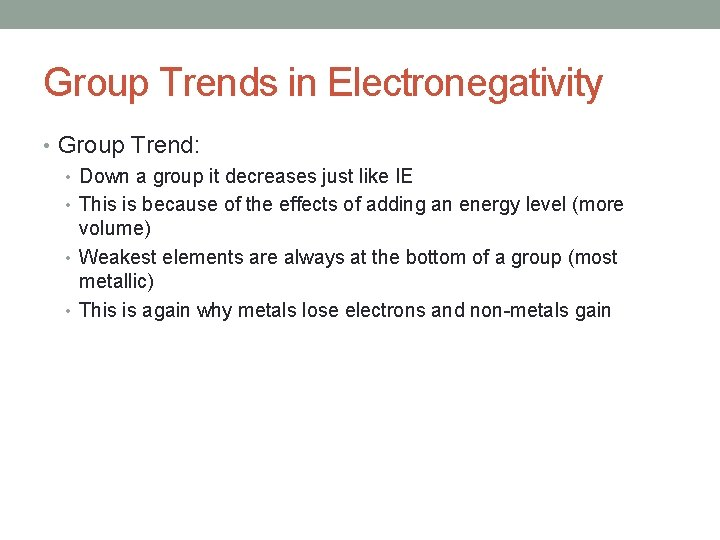Group Trends in Electronegativity • Group Trend: • Down a group it decreases just like IE • This is because of the effects of adding an energy level (more volume) • Weakest elements are always at the bottom of a group (most metallic) • This is again why metals lose electrons and non-metals gain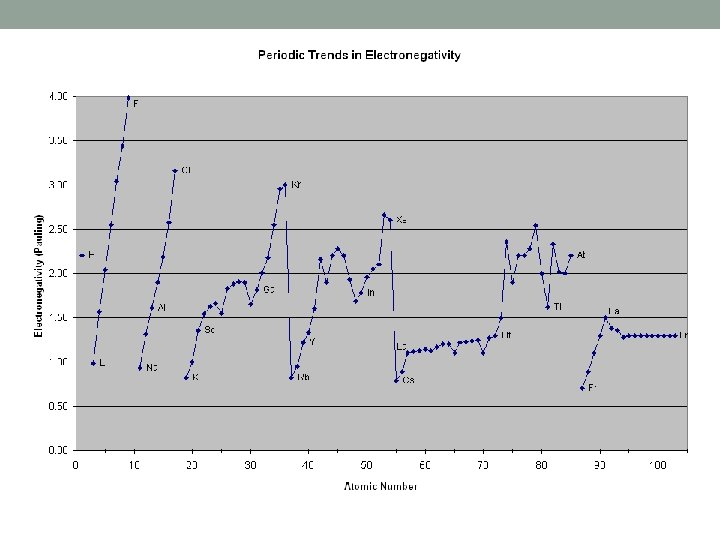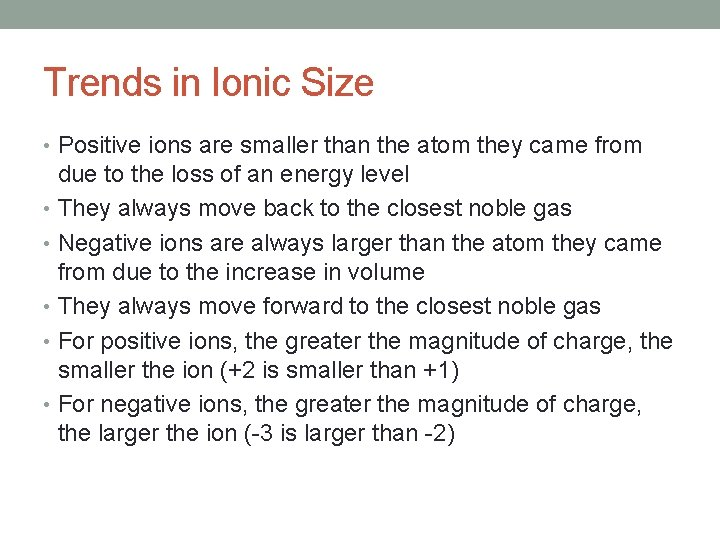Trends in Ionic Size • Positive ions are smaller than the atom they came from due to the loss of an energy level • They always move back to the closest noble gas • Negative ions are always larger than the atom they came from due to the increase in volume • They always move forward to the closest noble gas • For positive ions, the greater the magnitude of charge, the smaller the ion (+2 is smaller than +1) • For negative ions, the greater the magnitude of charge, the larger the ion (-3 is larger than -2)